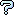All about flooble | fun stuff | Get a free chatterbox | Free JavaScript | Avatarsperplexus dot infoCubic Diophantine Conclusion (Posted on 2016-04-22)Each of A, B and C is a positive integer that satisfies this equation:

A3 + B3 = 31C3

Find the smallest value of A+B+C

 No Solution Yet Submitted by K Sengupta Rating: 4.0000 (1 votes)Comments: ( Back to comment list | You must be logged in to post comments.)re(2): Start| Comment 4 of 9 |(In reply to re: Start by broll)

From Google I learn that Fermat studied the curves x^3+y^3=Az^3 an stablish that if there is a non trivial solution then exists infinitely many.

Prestet working on Fermat get some formulas to obtain other solutions from one:

X=x(2y^3+x^3),  Y=-y(2x^3+y^3),  Z=z(x^3-y^3)

Using our solution (137, -65, 42) we get:
(277028111,  316425265,  119531076), all positive integers.

Then:
X+Y+Z= 712984452

and
31Z^3=5.2942468*10^25

I'm not sure this is the smallest value, but Charlie's search makes it quite possible.

Edited on April 23, 2016, 9:39 am
 Posted by armando on 2016-04-23 09:37:45Please log in:

 Search: Search body:
Forums (0)